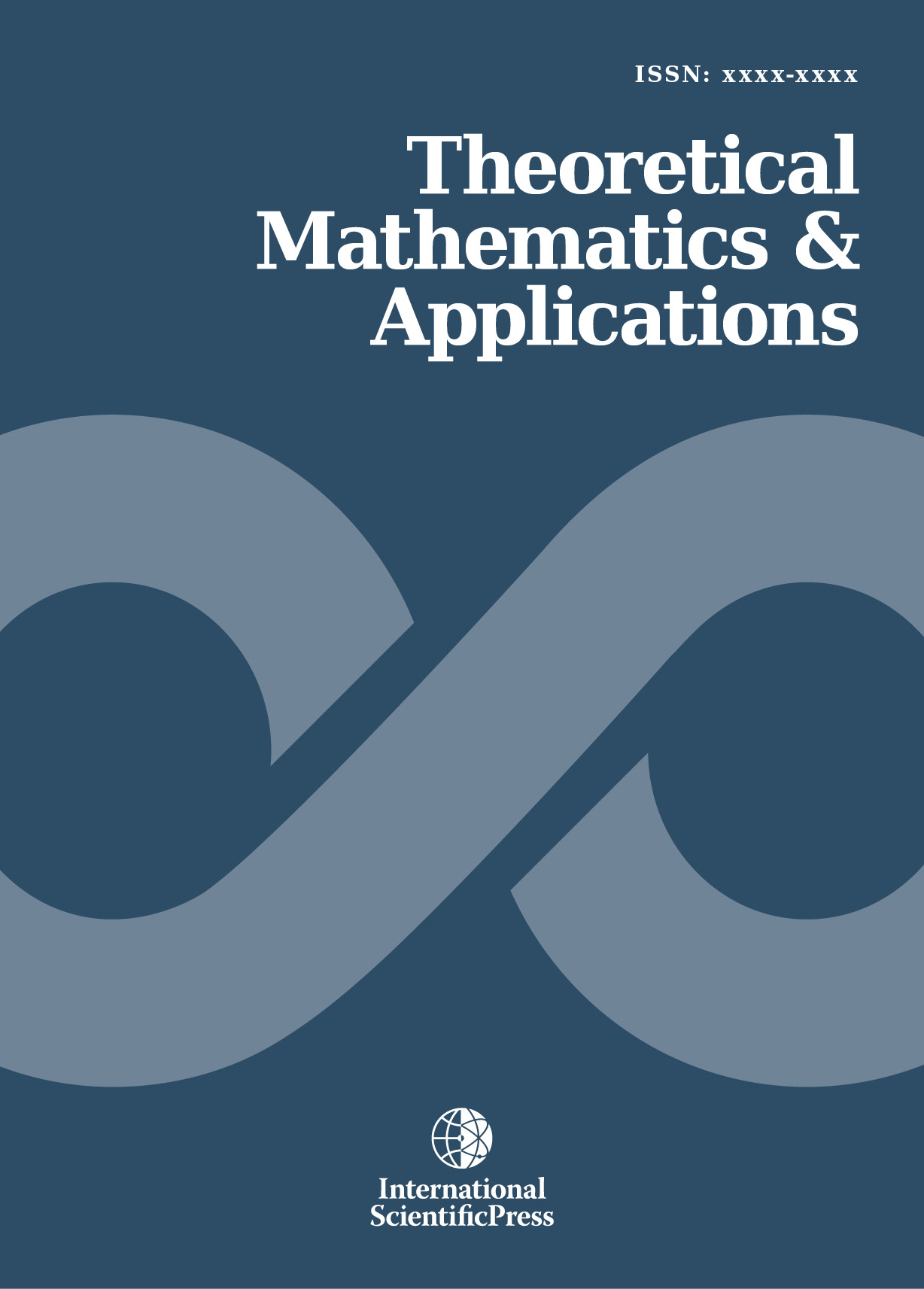# Theoretical Mathematics & Applications

#### Hidden properties of the Navier-Stokes equations.Double solutions. Origination of turbulence.

•[ Download ]
• Times downloaded: 946
•                                                     Abstract

The Navier-Stokes equations, which describe flows of fluids and gases, possess hidden properties that are discovered when studying the consistency of the conservation law equations involved into the set of  Navier- Stokes equations. Under such an investigation one obtains a nonidentical evolutionary relation for entropy as a state functional. This relation discloses peculiarities of the solutions to the Navier-Stokes equations due to which the Navier-Stokes equations can describe not only the change of physical quantities (such as energy, pressure, density) but also processes such as origination of waves, turbulent pulsations. From the evolutionary relation it follows that the Navier-Stokes equations possess solutions of two types, namely, the solution that is not a function and the solution that is a discrete function. The solutions of the first type are defined on nonintegrable manifold (like a tangent one) and describe the non-equilibrium state of a flow. And the solutions of the second type are defined on integrable structure and describe the locally equilibrium state of a flow. The transition from the solutions of the first type to ones of the second type describes the process of origination of turbulence. These results are obtained due to the skew-symmetric forms the basis of which are nonintegrable manifolds.ISSN: 1792-9687 (Print)
1792-9709 (Online)